Function Repository Resource:

# SampleRebalance

Resample data to correct for sampling bias

Contributed by: Jon McLoone
 ResourceFunction["SampleRebalance"][data,n] takes n samples from data weighted so that the first column of the result is uniformly distributed. ResourceFunction["SampleRebalance"][data,n,c] takes n samples from data weighted so that column c is uniformly distributed. ResourceFunction["SampleRebalance"][data,n,c,dist] takes n samples from data weighted so that column c is distributed according to dist.

## Details

ResourceFunction["SampleRebalance"] can be used to to correct for sampling bias by resampling the data so that a specific column of the data follows a presumed target distribution.
ResourceFunction["SampleRebalance"] assigns a weight to each record according to the PDF of the distribution evaluated at the value of the target column.
If an underspecified parametric distribution is provided for the distribution, its parameters are estimated from the target column data.
If data is given for the target distribution, that data is used to create a SmoothKernelDistribution.

## Examples

### Basic Examples (3)

A dataset:

 In:=Out=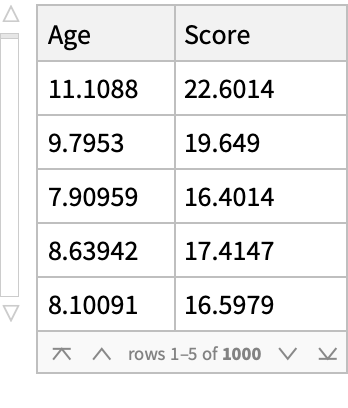Resample the data so that the "Age" column is uniformly distributed:

 In:=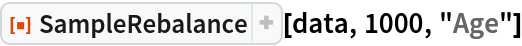Out=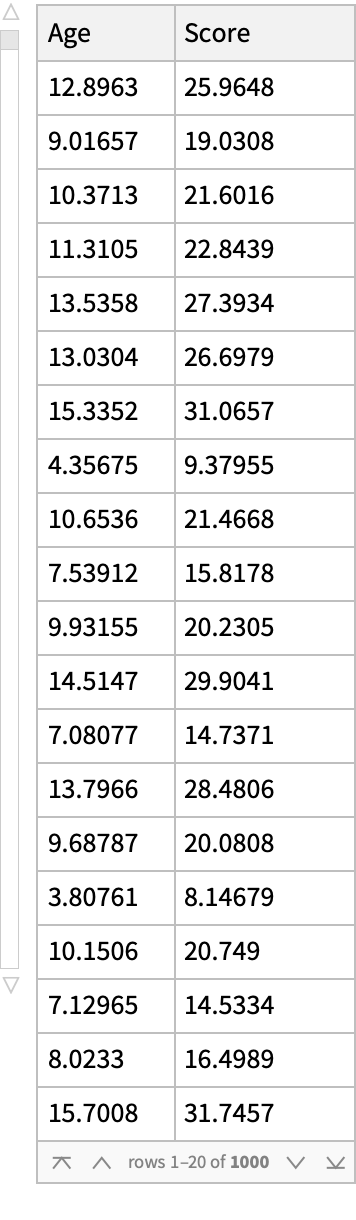Resample the data so that the "Age" column follows an Exponential[0.1] distribution:

 In:=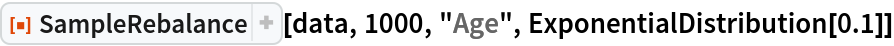Out=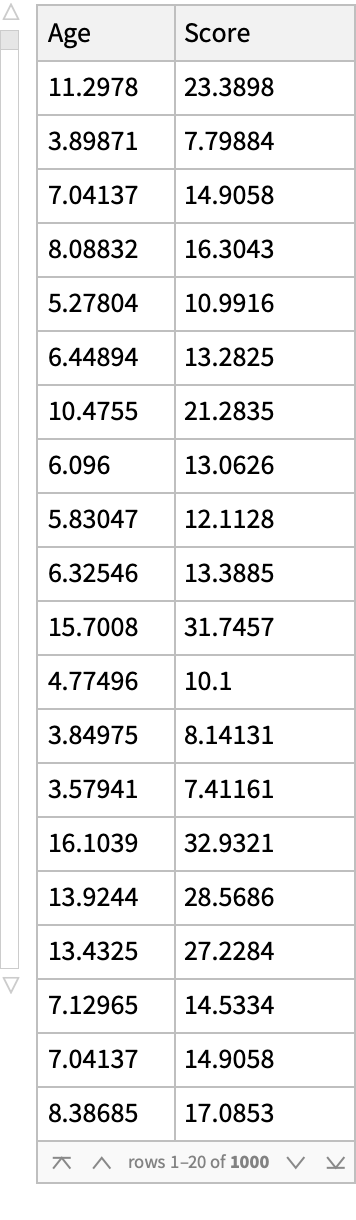### Scope (2)

A dataset:

 In:=Out=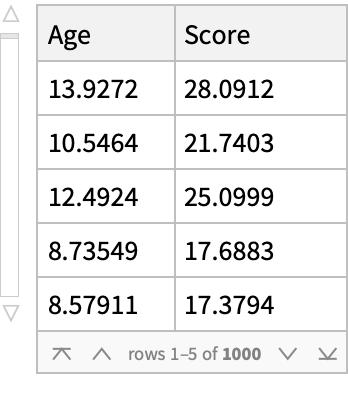Compute the mean of the data in the "Score" column:

 In:=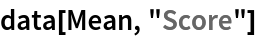Out=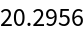The target distribution for "Age" can be given as a fully specified distribution:

 In:=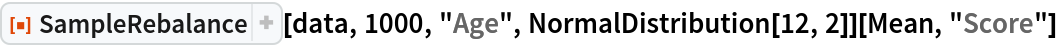Out=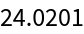One or more parameters can also be estimated from the "Age" data:

 In:=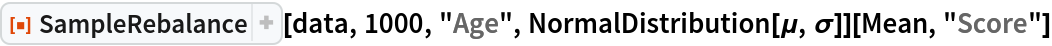Out=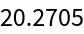A representative sample of "Age" values can also be provided:

 In:=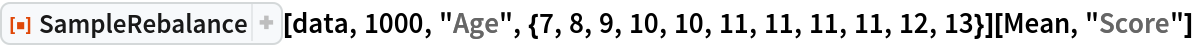Out=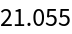The column being used for rebalancing can be specified by position instead of name:

 In:=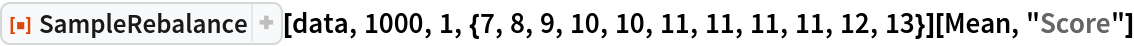Out=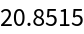Data can be provided as a Dataset of associations, a Dataset of lists or, as here, a List of lists:

 In:=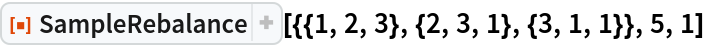Out=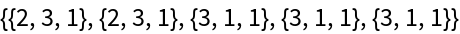If a column contains any non-numeric data, then it can be balanced against a CategoricalDistribution:

 In:=Out=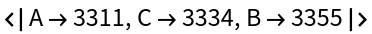Non-numeric reference data will be converted into a CategoricalDistribution:

 In:=Out=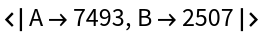### Applications (3)

In this synthetic dataset of children's test scores, it would appear that the scores are normally distributed:In:=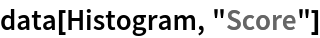Out=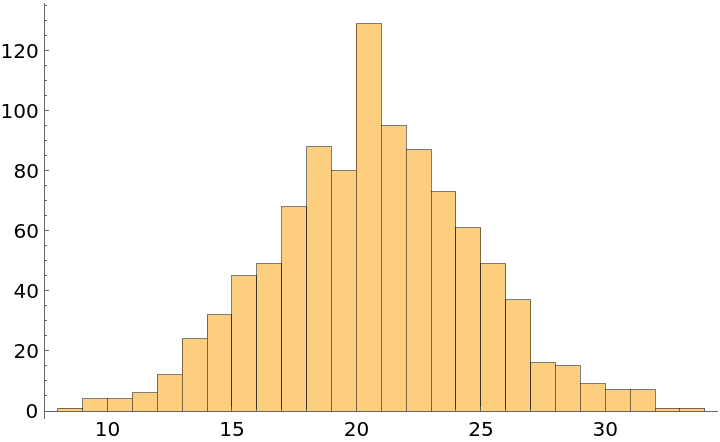However, this is because the data sample was biased toward 10-year-olds. If we assume that, across the whole population, all ages are equally represented, then the "Score" distribution appears more uniform:

 In:=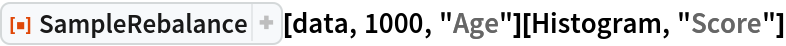Out=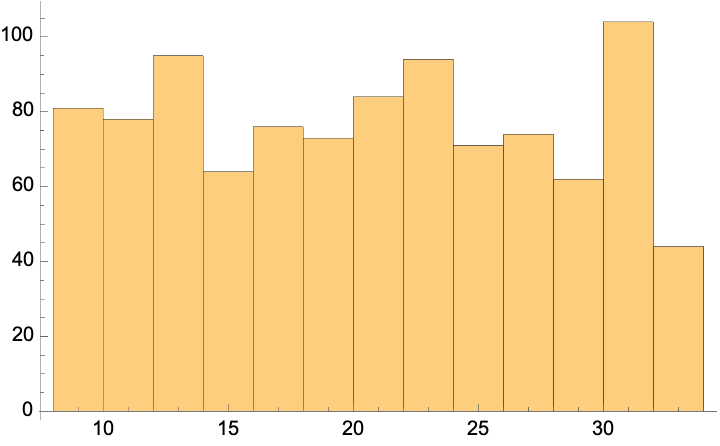If we assume that the ages of the population follow a certain ExponentialDistribution, then we see a different result:

 In:=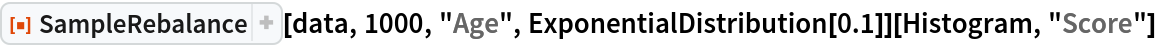Out=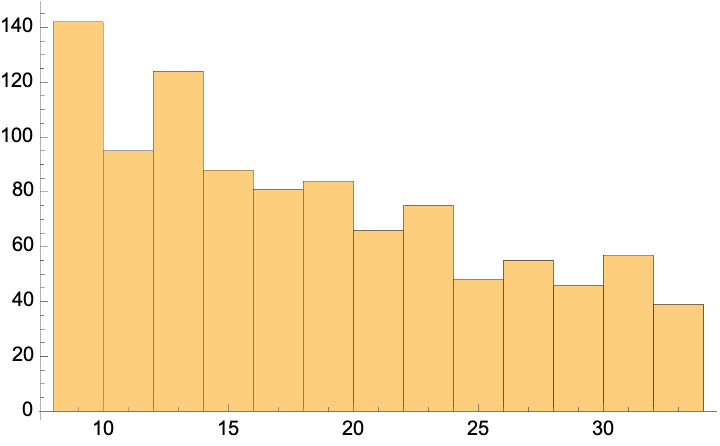### Properties and Relations (1)

After resampling the column used to rebalance it, the data should tend to the target distribution:In:=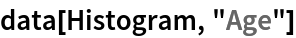Out=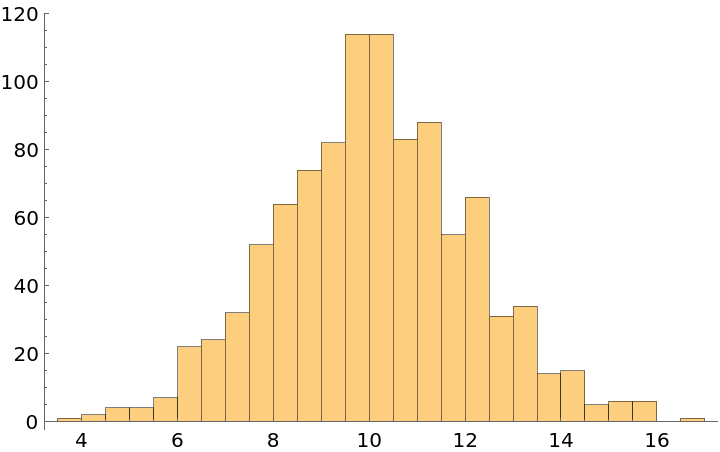In:=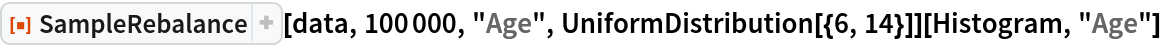Out=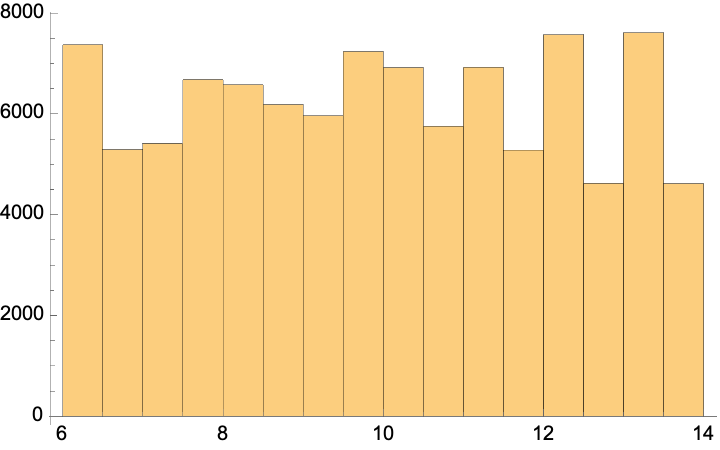### Possible Issues (1)

SampleRebalance only uses values from the original data and cannot always achieve the target reference distribution. In this example, there are no negative values, so the attempt to rebalance to NormalDistribution[0,5] results in the data distributed more like TruncatedDistribution[{0,},NormalDistribution[0,5]]:

 In:=Out=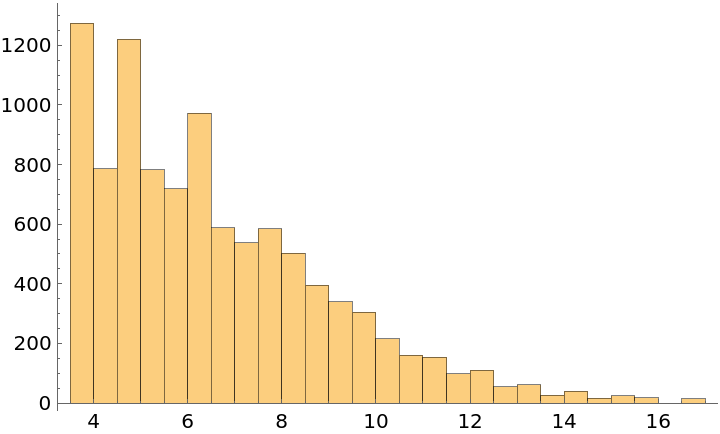Jon McLoone

## Version History

• 1.0.0 – 11 October 2021